# Feature tracking and optical flow¶

Faisal Qureshi
Professor
Faculty of Science
Ontario Tech University
http://vclab.science.ontariotechu.ca

In :
import numpy as np
import scipy as sp
from scipy import signal
import PIL as pil
import cv2 as cv
import matplotlib.pyplot as plt
from matplotlib import animation, rc, cm
from IPython.display import display, clear_output, HTML, Image


## Lesson plan¶

• Feature tracking
• Optical flow

## Motion cues¶

Human visual system is highly sensitive to motion.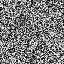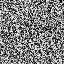## Recovering motion¶

• Feature tracking
• Extract visual features (corners, textured areas, etc.) and "track" them over multiple frames.
• Optical flow
• Recover image motion at each pixel from spatio-temporal image brightness variations (optical flow)

The two problems are closely related and can be studies in a common registration framework.

## Feature tracking¶

• An important part of many computer vision tasks. E.g., structure from motion techniques that attempt to estimate the metric structure of a scene using multiple images captured by a moving camera require matching points.
• If motion is small, tracking can be used to match points.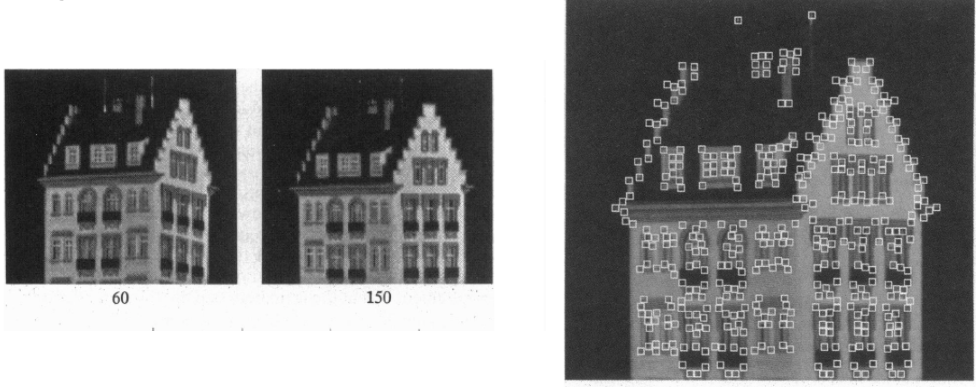## Challenges¶

• Find out which features can be tracked across frames – Tracking features efficiently – What happens if some points may change appearance over time (e.g., due to rotation, changing light conditions, etc.) – Small errors can accumulate as appearance model is updated
• This is often referred to as drift – Points may appear or disappear (say due to occlusions).
• Need to be able to add/delete tracked points

## Problem formulation¶

Given two frames at time $t$ and time $t+1$, estimate translation between a point in frame $t$ to its new location in frame $t+1$.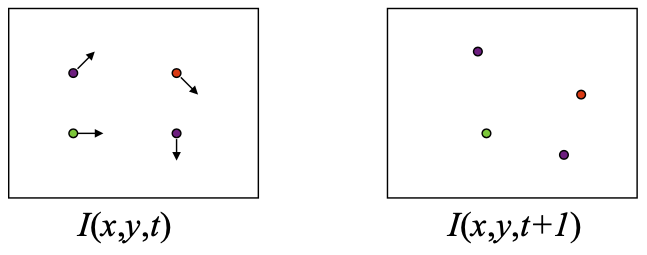B. Lucas and T. Kanade. An iterative image registration technique with an application to stereo vision. In Proceedings of the International Joint Conference on Artificial Intelligence, pp. 674–679, 1981.

• Brightness constancy
• Projection of the same point should "look" the same in every frame
• Small motion
• Points do not move far between frames at time $t$ and time $t+1$
• Spatial coherence
• Points move like their neighbours

## Brightness constancy equation¶

Consider a point at location $(x,y)$ in image at time $t$. This point moved to a new location $(x+u, y+v)$ in image at time $t+1$.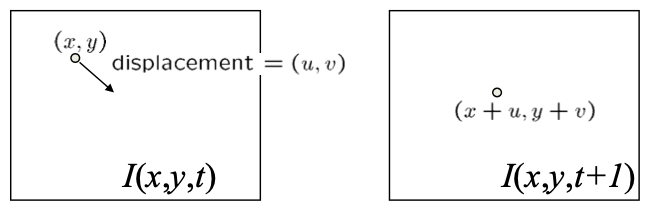Then the brightness-constancy equation is $$I(x,y,t) = I(x+u, y+v, t+1)$$

Lets take Taylor expansion of $I(x+u, y+v, t+1)$ at $(x,y,t)$

\begin{align} I(x+u, y+v, t+1) &= I(x,y,t) + uI_x + vI_y + I_t \\ \Rightarrow I(x+u, y+v, t+1) - I(x,y,t) &= uI_x + vI_y + I_t \end{align}

Here $I_x = \frac{\partial I}{\partial x}$, $I_y = \frac{\partial I}{\partial y}$, and $I_t = \frac{\partial I}{\partial t}$. Also, using the brightness-constancy assumption, the L.H.S. of the above equation is equal to 0.

\begin{align} u I_x + v I_y + I_t &= 0 \\ \Rightarrow \left[ \begin{array}{cc} I_x & I_y \end{array} \right] \left[ \begin{array}{c} u \\ v \end{array} \right] + I_t &= 0 \\ \Rightarrow \nabla I \left[ \begin{array}{c} u \\ v \end{array} \right] + I_t &= 0 \end{align}

## Aperture problem¶

Consider the figure shown below. $\nabla I$ is the (spatial) gradient at the pixel of interest. We know that gradient is normal to the edge. Say this pixel has optical flow equal to $(u,v)$. We can then re-write it as $$(u,v) = (u_e,v_e) + (u_p,v_p),$$ where $(u_e,v_e)$ represents the optical flow along the edge and $(u_p,v_p)$ represents the optical flow perpendicular to the edge.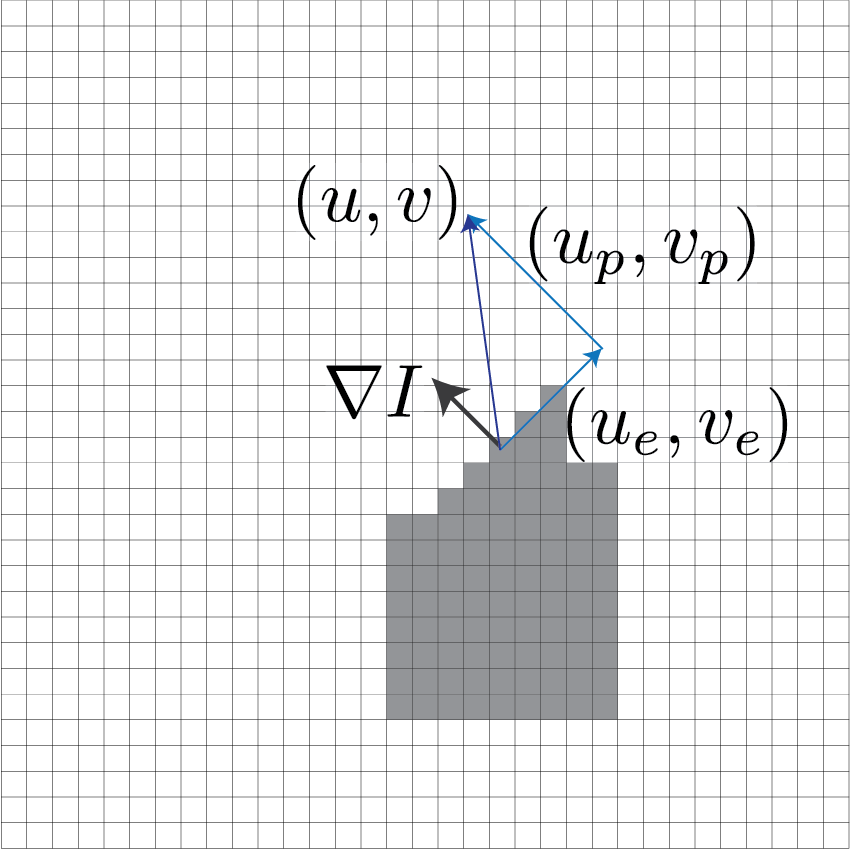Under this setup we can re-write the brightness constancy constraint equation as

\begin{align} \nabla I \left[ \begin{array}{c} u \\ v \end{array} \right] + I_t & = 0 \\ \Rightarrow \nabla I \left[ \begin{array}{c} u_e \\ v_e \end{array} \right] + \nabla I \left[ \begin{array}{c} u_p \\ v_p \end{array} \right] + I_t & = 0. \end{align}

We know that $\nabla I \perp [u_e, v_e]$, so $$\nabla I \left[ \begin{array}{c} u_e \\ v_e \end{array} \right]=0.$$

Therefore, we can re-write the equation as $$\nabla I \left[ \begin{array}{c} u_p \\ v_p \end{array} \right] • I_t = 0.$$

This means that the component of the motion perpendicular to the gradient (or along the edge) cannot be measured. This is referred to as the aperture problem.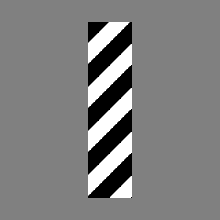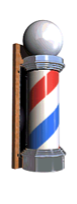Barber pole illusion

## Estimating motion $(u,v)$¶

How do we use the following equation to solve for $(u,v)$?

$$\nabla I \left[ \begin{array}{c} u \\ v \end{array} \right] + I_t = 0|$$

### Observations¶

• Two unknowns
• One equation per pixel
• Aperture problem

### Intuition¶

B. Lucas and T. Kanade. An iterative image registration technique with an application to stereo vision. In Proceedings of the International Joint Conference on Artificial Intelligence, pp. 674–679, 1981.

• Spatial coherence constraint
• Assume that pixel's neighbours have the same motion $(u,v)$.

$\newcommand{\bp}{\mathbf{p}_{#1}}$

E.g., if we use a 5-by-5 window that gives us 25 equations per pixel as seen below.

\begin{align} \left[ \begin{array}{cc} I_x(\bp{1}) & I_y(\bp{1}) \\ I_x(\bp{2}) & I_y(\bp{2}) \\ \vdots & \vdots \\ I_x(\bp{25}) & I_y(\bp{25}) \\ \end{array} \right] \left[ \begin{array}{c} u \\ v \end{array} \right] &= - \left[ \begin{array}{c} I_t(\bp{1}) \\ I_t(\bp{2}) \\ \vdots \\ I_t(\bp{25}) \\ \end{array} \right]. \end{align}

This is an over-constrained system of linear equations. We can solve it using least squares.

Let

$$\mathbf{A}=\left[ \begin{array}{cc} I_x(\bp{1}) & I_y(\bp{1}) \\ I_x(\bp{2}) & I_y(\bp{2}) \\ \vdots & \vdots \\ I_x(\bp{25}) & I_y(\bp{25}) \\ \end{array} \right] \mathrm{,\ } \mathbf{d}= \left[ \begin{array}{c} u \\ v \end{array} \right] \mathrm{\ and\ } \mathbf{b} = - \left[ \begin{array}{c} I_t(\bp{1}) \\ I_t(\bp{2}) \\ \vdots \\ I_t(\bp{25}) \\ \end{array} \right]$$

Then we can find $\mathbf{d}$ as follows:

\begin{align} \mathbf{A} \mathbf{d} &= \mathbf{b} \\ \Rightarrow \mathbf{A}^T \mathbf{A} \mathbf{d} &= \mathbf{A}^T \mathbf{b} \\ \Rightarrow (\mathbf{A}^T \mathbf{A})^{-1} (\mathbf{A}^T \mathbf{A}) \mathbf{d} &= (\mathbf{A}^T \mathbf{A})^{-1} \mathbf{A}^T \mathbf{b} \\ \Rightarrow \mathbf{d} &= (\mathbf{A}^T \mathbf{A})^{-1} \mathbf{A}^T \mathbf{b} \end{align}

Note that in general $\mathbf{A}$ is not a square matrix, so we cannot compute its inverse. Instead, we compute its psuedo-inverse $(\mathbf{A}^T \mathbf{A})^{-1}$. For more details check out the notes on line fitting.

## Relationship to corner detection¶

Note that

$$\mathbf{A}^T \mathbf{A} = \left[ \begin{array}{cc} \sum I_x I_x & \sum I_x I_y \\ \sum I_x I_y & \sum I_y I_y \\ \end{array} \right] = \sum \left[ \begin{array}{cc} I^2_x & I_x I_y \\ I_x I_y & I^2_y \\ \end{array} \right]$$

In order to estimate $\mathbf{d}$, the following should hold:

• $\mathbf{A}^T \mathbf{A}$ should be invertible
• $\mathbf{A}^T \mathbf{A}$ should not be too small to avoid numerical issues
• i.e., eigenvalues $\lambda_1$ and $\lambda_2$ of $\mathbf{A}^T \mathbf{A}$ should not be too small (no flat region)
• $\mathbf{A}^T \mathbf{A}$ should not be ill-conditioned
• i.e., the ratio of the larger to the smaller eigenvalue should not be too large (no edge)

Exactly the same as the criteria for Harris corner detector.

Even without relying upon the knowledge of linear algebra, note that $\mathbf{A}^T \mathbf{A}$ is the second moment matrix that we use to detect Harris corners.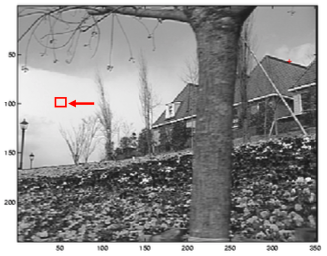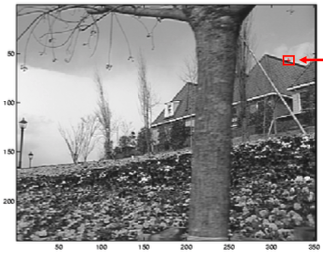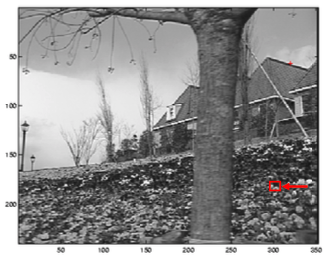(Left to right) flat region, gradients have small magnitudes, $\lambda_1$ and $\lambda_2$ are both very small; edge region, one gradient is very small and the other gradient is very large, large $\lambda_1$ and small $\lambda_2$; textured region, gradients are large, both $\lambda_1$ and $\lambda_2$ are large.

## Actual and percieved motion¶

Notice how the presence of a corner in the figure on the right enables us to see left-to-right motion of the line. The figure on the left other hand doesn't have any such cue, and the motion appears to be along the right-up diagonal. The actual motion is from left-to-right.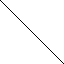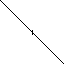## Dealing with large motions¶

Recall that we used Taylor series expansion to construct the brighness constancy constaint equation. The implicit assumption being pixel motion $(u,v)$ is small between frames at time $t$ and at time $t+1$. This suggests that as formulated, our approach will perform poorly only large motions. We can handle large motions via iterative refinement.

1. Initialize $(x',y')=(x,y)$
2. Compute $(u,v)$ using the method discussed above
3. Shift window by $(u,v)$, i.e., compute new $(x',y') = (x'+u, y'+v)$
• Need subpixel values that can be computed via interpolation
4. Recalculate $I_t$
5. Repeat steps 1 to 4 until converges

### Course-to-fine registration¶

Use image pyramids for frame at time $t$ and frame at time $t+1$ to compute optical flow in a course-to-fine fashion.

• Compute optical flow at coarser levels (lower res) first first and use those values to set up the optical flow estimation problem at finer levels (higher res).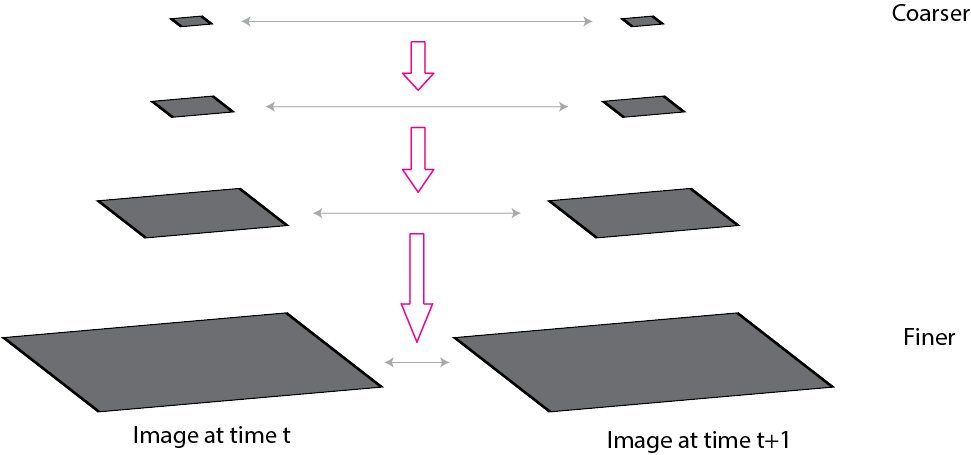## Shi-Tomasi feature tracker¶

J. Shi and C. Tomasi. Good Features to Track. CVPR 1994.

• Find good features using eigenvalues of second-moment matrix (e.g., Harris detector or threshold on the smallest eigenvalue) – “good” features to track are the ones whose motion can be estimated reliably
• Track from frame to frame with Lucas-Kanade – This amounts to assuming a translation model for frame-to-frame feature movement
• Check consistency of tracks by affine registration to the first observed instance of the feature – Affine model is more accurate for larger displacements – Comparing to the first frame helps to minimize drift

### Tracking example¶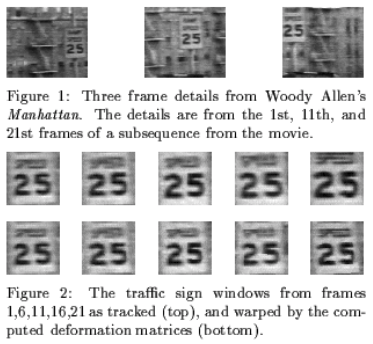## Implementation issues¶

• small window more sensitive to noise and may miss larger motions (without pyramid)
• large window more likely to cross an occlusion boundary (and it’s slower)
• 15-by-15 to 31-by-31 seems typical
• common to apply weights so that center matters more (e.g., with Gaussian)

## Perception of motion¶

Even "improvished" motion data can evoke a strong percept

G. Johansson, “Visual Perception of Biological Motion and a Model For Its Analysis", Perception and Psychophysics 14, 201-211, 1973.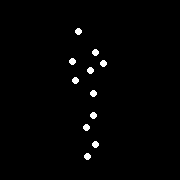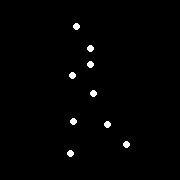## Uses of motion¶

• Estimating 3D structure
• Segmenting objects based on motion cues
• Learning and tracking dynamical models
• Recognizing events and activities
• Improving video quality (motion stabilization)

## Motion field¶

Definition: the projection of the 3D scene motion into the image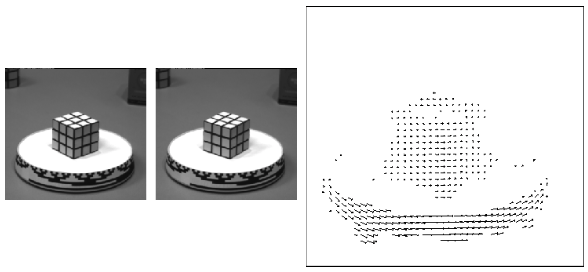Question: what would the motion field of a non-rotating ball moving towards the camera look like?

## Optical flow¶

Definition: optical flow is the apparent motion of brightness patterns in the image.

Ideally, optical flow would be the same as the motion field, however, apparent motion can be caused by lighting changes without any actual motion. E.g., think of a uniform rotating sphere under fixed lighting vs. a stationary sphere under moving illumination.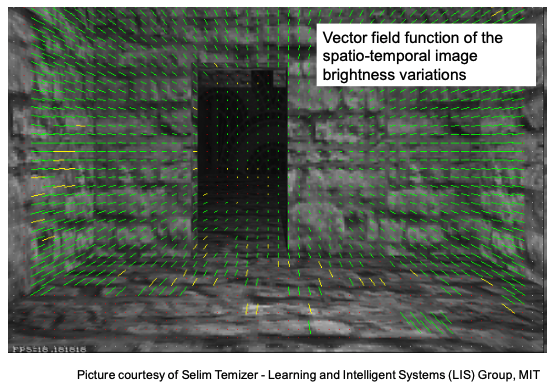• same as Lucas-Kanade feature tracking, but for each pixel
• works better for textured pixels
• to improve efficiency, operations can be done one frame at a time rather than pixel by pixel

1. Compute "simple" LK at highest level
2. At level $i$
• Take flow $u_{i-1},v_{i-1}$ from level $i-1$
• bilinear interpolate it to create $u_i^*, v_i^*$ matrices of twice resolution for level $i$
• multiply $u_i^*, v_i^*$ by $2$
• compute $f_t$ from a block displaced by $u_i^*(x,y),v_i^*(x,y)$
• apply LK to get $u'_i(x,y),v'_i(x,y)$ (the correction in flow)
• update: $u_i = u_i^* + u'_i$ and $v_i = v_i^* + v'_i$

1. estimate displacement at each pixel by solving Lucas-Kanade equations
2. warp $I(t)$ towards $I(t+1)$ using the estimated flow field
• interpolation
3. repeat until convergence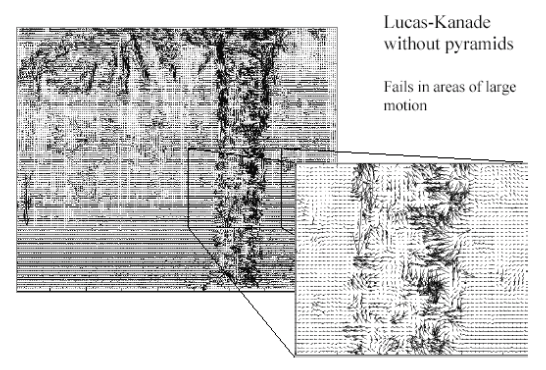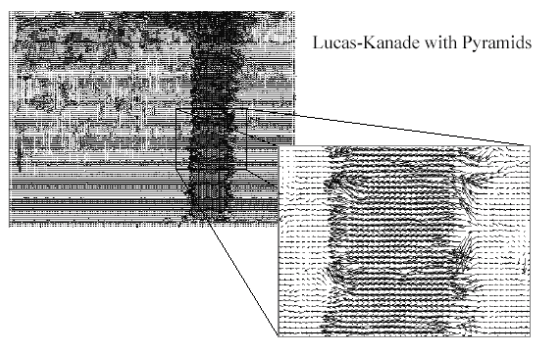Left to right. LK optical flow with and without pyramids. (From Khurram H. Shafique.)

## Sources of errors in Lucas-Kanade optical flow¶

1. The motion is large
• Possible Fix: Keypoint matching
2. A point does not move like its neighbors
• Possible Fix: Region-based matching
3. Brightness constancy does not hold

## Improving optical flow (before deep learning)¶

Add one or more to LK

• energy minimization with smoothing term
• region matching
• keypoint matching (long-range)

Large displacement optical flow, Brox et al., CVPR 2009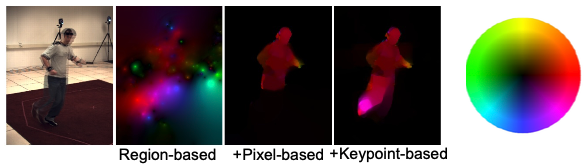The above ideas can also be explored when designing deep learning methods for computing optical flow.

Aside: we can use color encodings to display optical flow, similar to how we visualized gradients.

## Summary¶

• Major contributions from Lucas, Tomasi and Kanade

• Feature tracking
• Optical flow
• Key idea

• By assuming brightness constancy, truncated Taylor expansion leads to simple and fast patch matching across frames
• Course-to-fine registrationIn [ ]: